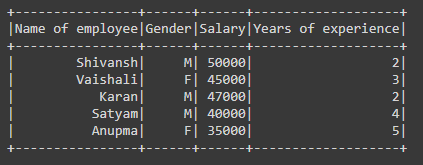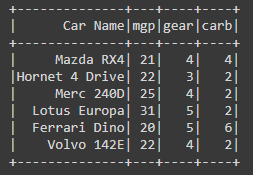Related Articles

# How to create PySpark dataframe with schema ?

• Difficulty Level : Hard
• Last Updated : 09 May, 2021

In this article, we will discuss how to create the dataframe with schema using PySpark. In simple words, the schema is the structure of a dataset or dataframe.

### Functions Used:

For creating the dataframe with schema we are using:

Syntax: spark.createDataframe(data,schema)

Parameter:

• data – list of values on which dataframe is created.
• schema – It’s the structure of dataset or list of column names.

where spark is the SparkSession object.

Example 1:

• In the below code we are creating a new Spark Session object named ‘spark’.
• Then we have created the data values and stored them in the variable named ‘data’ for creating the dataframe.
• Then we have defined the schema for the dataframe and stored it in the variable named as ‘schm’.
• Then we have created the dataframe by using createDataframe() function in which we have passed the data and the schema for the dataframe.
• As dataframe is created for visualizing we used show() function.

## Python

 `# importing necessary libraries``from` `pyspark.sql ``import` `SparkSession`` ` `# function to create new SparkSession``def` `create_session():``  ``spk ``=` `SparkSession.builder \``      ``.master(``"local"``) \``      ``.appName(``"Geek_examples.com"``) \``      ``.getOrCreate()``  ``return` `spk`` ` `# main function``if` `__name__ ``=``=` `"__main__"``:`` ` `  ``# calling function to create SparkSession``  ``spark ``=` `create_session()`` ` `  ``#  creating data for creating dataframe ``  ``data ``=` `[``    ``(``"Shivansh"``,``"M"``,``50000``,``2``),``    ``(``"Vaishali"``,``"F"``,``45000``,``3``),``    ``(``"Karan"``,``"M"``,``47000``,``2``),``    ``(``"Satyam"``,``"M"``,``40000``,``4``),``    ``(``"Anupma"``,``"F"``,``35000``,``5``)``  ``]`` ` `  ``# giving schema``  ``schm``=``[``"Name of employee"``,``"Gender"``,``"Salary"``,``"Years of experience"``]`` ` `  ``# creating dataframe using createDataFrame()``  ``# function in which pass data and schema``  ``df ``=` `spark.createDataFrame(data,schema``=``schm)`` ` `  ``# visualizing the dataframe using show() function``  ``df.show()`

Output:Example 2:

In the below code we are creating the dataframe by passing data and schema in the createDataframe() function directly.

## Python

 `# importing necessary libraries``from` `pyspark.sql ``import` `SparkSession`` ` `# function to create new SparkSession``def` `create_session():``  ``spk ``=` `SparkSession.builder \``      ``.master(``"local"``) \``      ``.appName(``"Geek_examples.com"``) \``      ``.getOrCreate()``  ``return` `spk`` ` `# main function``if` `__name__ ``=``=` `"__main__"``:`` ` `  ``# calling function to create SparkSession``  ``spark ``=` `create_session()`` ` `  ``# creating dataframe using createDataFrame() ``  ``# function in which pass data and schema``  ``df ``=` `spark.createDataFrame([``    ``(``"Mazda RX4"``,``21``,``4``,``4``),``    ``(``"Hornet 4 Drive"``,``22``,``3``,``2``),``    ``(``"Merc 240D"``,``25``,``4``,``2``),``    ``(``"Lotus Europa"``,``31``,``5``,``2``),``    ``(``"Ferrari Dino"``,``20``,``5``,``6``),``    ``(``"Volvo 142E"``,``22``,``4``,``2``)``  ``],[``"Car Name"``,``"mgp"``,``"gear"``,``"carb"``])`` ` `  ``# visualizing the dataframe using show() function``  ``df.show()`

Output:Attention geek! Strengthen your foundations with the Python Programming Foundation Course and learn the basics.

To begin with, your interview preparations Enhance your Data Structures concepts with the Python DS Course. And to begin with your Machine Learning Journey, join the Machine Learning – Basic Level Course

My Personal Notes arrow_drop_up